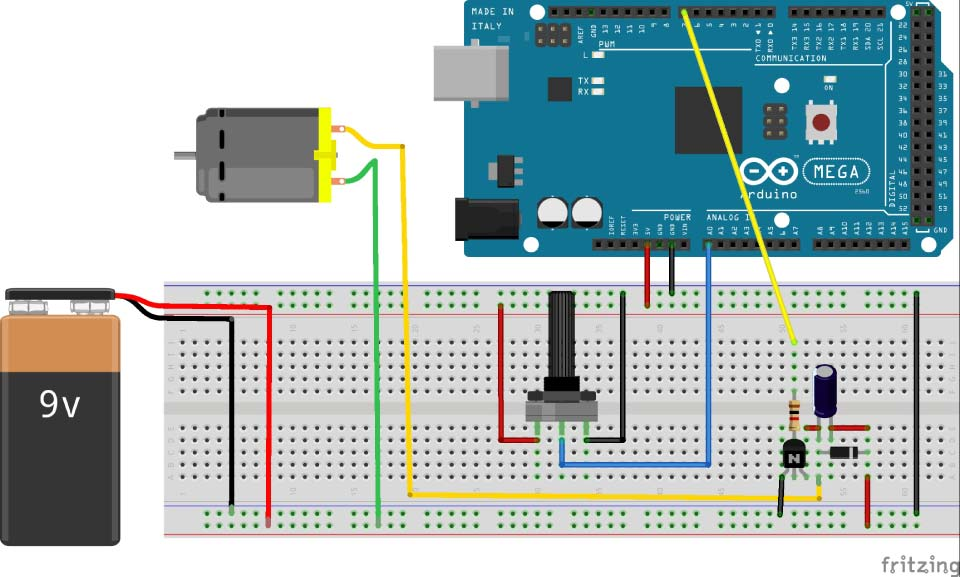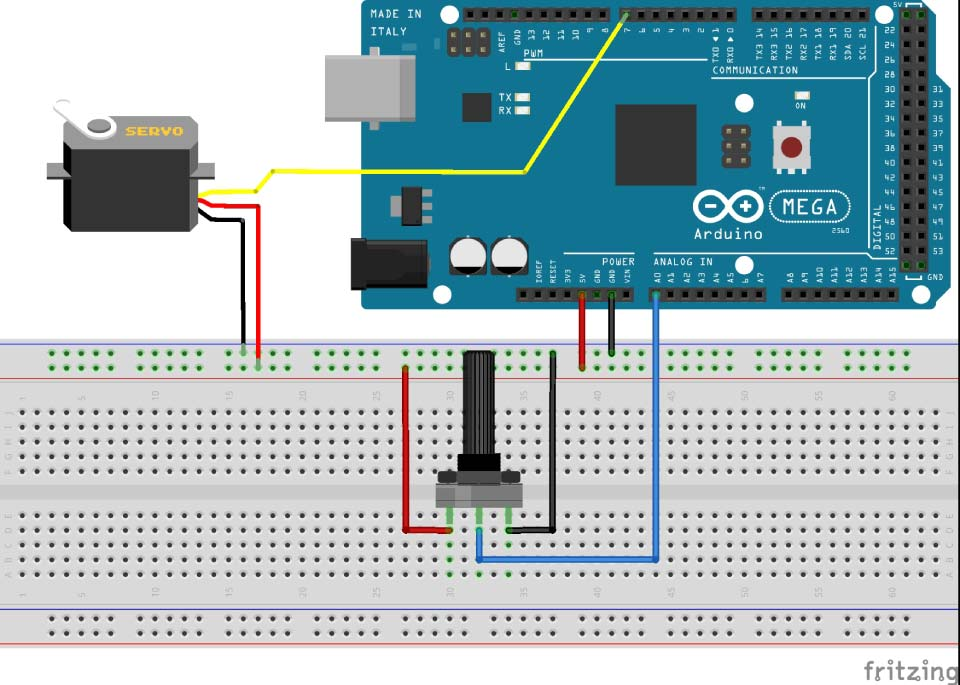# Arduino Tutorial 04: Motors

Welcome to the fourth Arduino Tutorial from our Arduino Tutorial Series. In this tutorial we will learn how to control DC and Servo Motors using PWM (Pulse Width Modulation).

This is a Step by Step Video Tutorial which is easy to be followed. Also, below the video you can find what Parts do we need for this tutorial and the Source Codes of the Examples in the video.

Components needed for the first example

Disclosure: These are affiliate links. As an Amazon Associate I earn from qualifying purchases.

### Circuit schematic of the first example, controlling a DC Motor### Source Code of the first example, controlling a DC Motor

```int pwmPin = 7;

void setup() {
Serial.begin(9600);
pinMode( pwmPin, OUTPUT);
}

void loop () {

int newpotValue = map(potValue, 0, 1023, 0 , 255);

analogWrite(pwmPin, newpotValue);
}```

### Parts needed for the second example, controlling a Servo Motor

• Servo Motor
• Potentiometer

### Circuit schematic of the second example, controlling a Servo Motor### Source Code of the second example, controlling a Servo Motor

```#include <Servo.h>

Servo myServo;

void setup() {

myServo.attach(7);
}

void loop() {